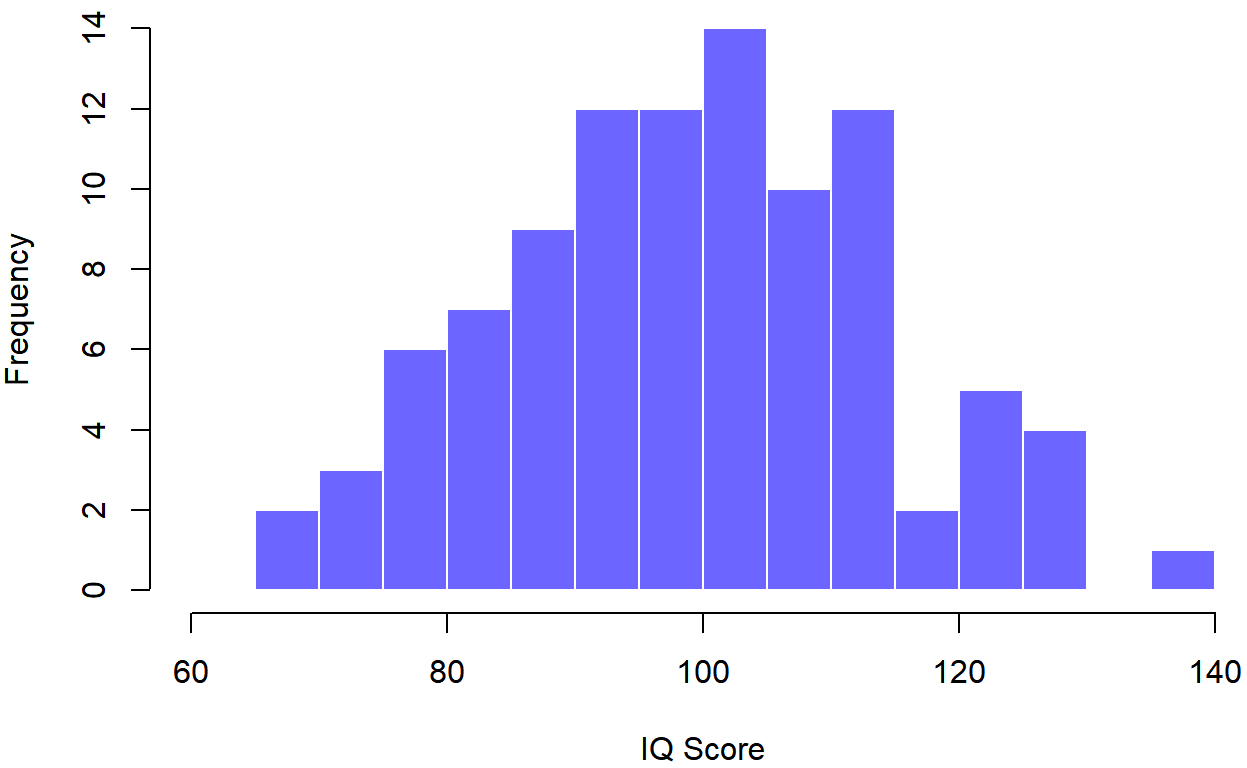# 7.2: Samples and Populations Refresher

•• Michelle Oja
• Taft College
$$\newcommand{\vecs}{\overset { \rightharpoonup} {\mathbf{#1}} }$$ $$\newcommand{\vecd}{\overset{-\!-\!\rightharpoonup}{\vphantom{a}\smash {#1}}}$$$$\newcommand{\id}{\mathrm{id}}$$ $$\newcommand{\Span}{\mathrm{span}}$$ $$\newcommand{\kernel}{\mathrm{null}\,}$$ $$\newcommand{\range}{\mathrm{range}\,}$$ $$\newcommand{\RealPart}{\mathrm{Re}}$$ $$\newcommand{\ImaginaryPart}{\mathrm{Im}}$$ $$\newcommand{\Argument}{\mathrm{Arg}}$$ $$\newcommand{\norm}{\| #1 \|}$$ $$\newcommand{\inner}{\langle #1, #2 \rangle}$$ $$\newcommand{\Span}{\mathrm{span}}$$ $$\newcommand{\id}{\mathrm{id}}$$ $$\newcommand{\Span}{\mathrm{span}}$$ $$\newcommand{\kernel}{\mathrm{null}\,}$$ $$\newcommand{\range}{\mathrm{range}\,}$$ $$\newcommand{\RealPart}{\mathrm{Re}}$$ $$\newcommand{\ImaginaryPart}{\mathrm{Im}}$$ $$\newcommand{\Argument}{\mathrm{Arg}}$$ $$\newcommand{\norm}{\| #1 \|}$$ $$\newcommand{\inner}{\langle #1, #2 \rangle}$$ $$\newcommand{\Span}{\mathrm{span}}$$

## Population Parameters and Sample Statistics

Up to this point we have been talking about populations the way a scientist might. To a psychologist, a population might be a group of people. To an ecologist, a population might be a group of bears. In most cases the populations that scientists care about are concrete things that actually exist in the real world. Statisticians, however, are a funny lot. On the one hand, they are interested in real world data and real science in the same way that scientists are. On the other hand, they also operate in the realm of pure abstraction in the way that mathematicians do. As a consequence, statistical theory tends to be a bit abstract in how a population is defined. Statisticians operationalize the concept of a “population” in terms of mathematical objects that they know how to work with, namely: probability distributions.

The idea is quite simple. Let’s say we’re talking about IQ scores. To a psychologist, the population of interest is a group of actual humans who have IQ scores. A statistician “simplifies” this by operationally defining the population as the probability distribution depicted in Figure $$\PageIndex{1}$$. IQ tests are designed so that the average IQ is 100, the standard deviation of IQ scores is 15, and the distribution of IQ scores is normal. These values are referred to as the population parameters because they are characteristics of the entire population. That is, we say that the population mean μ (mu) is 100, and the population standard deviation is 15. [Although not directly related to this conversation, we also covered non-parametric analyses in Ch. 4.5, which were analyses that we could do when we don't think that the population is normally distributed.]Figure $$\PageIndex{1}$$- Probability Distribution of IQ (CC-BY-SA- Danielle Navarro from Learning Statistics with R)

Now suppose I run an experiment. I select 100 people at random and administer an IQ test, giving me a random sample from the population. Each of these IQ scores is sampled from a normal distribution with mean 100 and standard deviation 15. So if I plot a histogram of the sample, I get something like the one shown in Figure $$\PageIndex{2}$$.Figure $$\PageIndex{2}$$- Probability Distribution of 100 IQ Scores (CC-BY-SA- Danielle Navarro from Learning Statistics with R)

As you can see, the histogram is roughly the right shape, but it’s a very crude approximation to the true population distribution shown in Figure $$\PageIndex{1}$$. Even though there seems to be an outlier who scored near 140 points on the IQ test, the mean of my sample is fairly close to the population mean 100, but not identical. In this case, it turns out that the people in my sample have a mean IQ of 98.5 ($$\displaystyle \bar{X} = 98.5$$), and the standard deviation of their IQ scores is 15.9. These sample statistics are descriptive of the data set, and although they are fairly similar to the true population values, they are not the same the population parameters. In general, sample statistics are the things you can calculate from your data set, and the population parameters are the things you want to learn about.

As we learned in the chapter on distributions, the bigger the sample, the more like a normal curve is is. You can see this in Figure $$\PageIndex{3}$$, a random selection of 10,000 IQ scores from a regular population looks very much like a normal distribution.Figure $$\PageIndex{3}$$- Probability Distribution of 10,000 IQ Scores (CC-BY-SA- Danielle Navarro from Learning Statistics with R)

## Onward and upward!

The next section talks a little more about how samples are sometimes similar to their population, and sometimes not...

7.2: Samples and Populations Refresher is shared under a CC BY-SA 4.0 license and was authored, remixed, and/or curated by Michelle Oja.